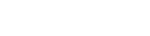# Cool algorithm for binary text string

Today’s progress was about bug fixing the block parts such as Register, Shift Register and Counter.

I simplified their code and had an idea for a better way to display number values. Basically, the default is 8-bit data, but it may be up to 16-bit which allows for when we do arithmetic such as multiplication. And we may set a preference for 4-bit nibbles, yet have the size expand out to accommodate larger numbers when displaying them for a Bus for example.

This is easy to do with say a hex format string such as `0x%02X` which will show at least 2 significant digits, but will expand out to 4 digits if need be.

For the binary text function I came up with this code:

``````# Create groups of 4 bits
func int2bin(x: int, num_bits = 8) -> String:
var _b = ""
for n in 16:
if n > 0 and n % 4 == 0:
if x == 0 and n == num_bits:
break
_b = " " + _b
_b = String(x % 2) + _b
x /= 2
return "0b" + _b
``````

This groups binary digits into space-separated chunks of 4 digits and has a minimum bit length.

Have to test the ALU block next.

## More Devlog entries

Most recent first